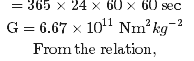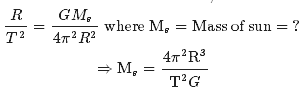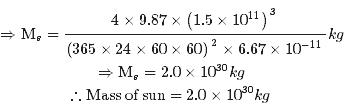Courses

# Test: Gravitation- 1

## 20 Questions MCQ Test Physics Class 11 | Test: Gravitation- 1

Description
This mock test of Test: Gravitation- 1 for JEE helps you for every JEE entrance exam. This contains 20 Multiple Choice Questions for JEE Test: Gravitation- 1 (mcq) to study with solutions a complete question bank. The solved questions answers in this Test: Gravitation- 1 quiz give you a good mix of easy questions and tough questions. JEE students definitely take this Test: Gravitation- 1 exercise for a better result in the exam. You can find other Test: Gravitation- 1 extra questions, long questions & short questions for JEE on EduRev as well by searching above.
QUESTION: 1

### According to Kepler’s Law of orbits:

Solution:
• The orbit of a planet around the Sun (or of a satellite around a planet) is not a perfect circle. It is an ellipse—a “flattened” circle.
• The Sun (or the centre of the planet) occupies one focus of the ellipse. A focus is one of the two internal points that help determine the shape of an ellipse.
• The distance from one focus to any point on the ellipse and then back to the second focus is always the same.

Kepler's Law of Orbits:QUESTION: 2

### Escape speed from the earth is the

Solution:

The escape velocity is the minimum velocity required to leave a planet or moon. For a rocket or other object to leave a planet, it must overcome the pull of gravity.

Vescape = √2GM/R
Vescape = 11184 m/sec approximate to 11.2 km/sec

QUESTION: 3

### According to Kepler’s Law of areas,

Solution:
• Kepler law of areas describes the speed of a planet travelling in an elliptical orbit around the sun. A planet’s orbital speed changes, depending on how far it is from the Sun.
• The closer a planet is to the Sun, the stronger the Sun’s gravitational pull on it, and the faster the planet moves.
• The farther it is from the Sun, the weaker the Sun’s gravitational pull, and the slower it moves in its orbit.
• So, statement of Kepler's law of area is "The line joining the sun to the planet sweeps out equal areas in equal interval of time". i.e. areal velocity is constant.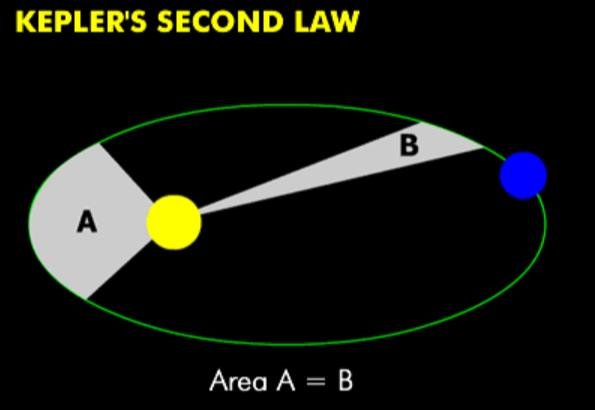QUESTION: 4

The total energy of a circularly orbiting satellite is

Solution:
• When a satellite revolves around a planet in its orbit, it possesses both potential energy (due to its position against the gravitational pull of earth) and kinetic energy (due to orbital motion).
• The total energy of a circularly orbiting satellite is thus negative, with the potential energy being negative but twice is the magnitude of the positive kinetic energy.
QUESTION: 5

According to Kepler’s Law of periods, The  _______________ of the time period of revolution of a planet is proportional to the cube of the  ___________ of the ellipse traced out by the planet

Solution:

Kepler's 3rd law is a mathematical formula. It means that if you know the period of a planet's orbit (T = how long it takes the planet to go around the Sun), then you can determine that planet's distance from the Sun (a is the length of the semimajor axis of the planet's orbit)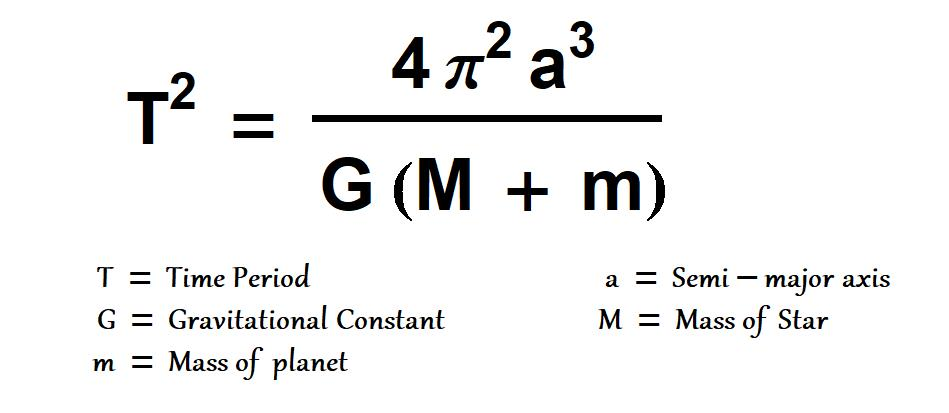QUESTION: 6

A ‘central’ force is always directed

Solution:
• The motion of a particle under a central force F always remains in the plane defined by its initial position and velocity. This may be seen by symmetry.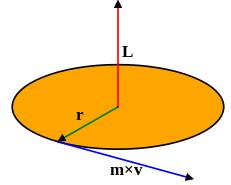• Since the position r, velocity v and force F all lie in the same plane, there is never an acceleration perpendicular to that plane, because that would break the symmetry between "above" the plane and "below" the plane.
• To demonstrate this mathematically, it suffices to show that the angular momentum of the particle is constant. This angular momentum L is defined by the equation r×r×mv
QUESTION: 7

The acceleration due to gravity at the North Pole of Neptune is approximately 10.7 m/s2. Neptune has mass 1.0 x 1026 kg and radius 2.5 x 104 km and rotates once around its axis in about 16 h. What is the gravitational force on a 5.0-kg object at the north pole of Neptune?

Solution:

Gravitational Force:
F = mg
⇒ F = 5×10.7 = 53.5 N

QUESTION: 8

To find the resultant gravitational force acting on the particle m due to a number of masses we need to use:

Solution:

According to properties of gravitational force, gravitational force between the particles is independent of the presence or absence of other particles; so the principle of superposition is valid i.e. force on a particle due to number of particles is the resultant of forces due to individual particles.

QUESTION: 9

For Geostationary Satellites

Solution:

The satellite which appear stationary relative to earth, such satellites are called geostationary satellites and will have a period of rotation as the period of rotation of earth i.e  24 Hrs.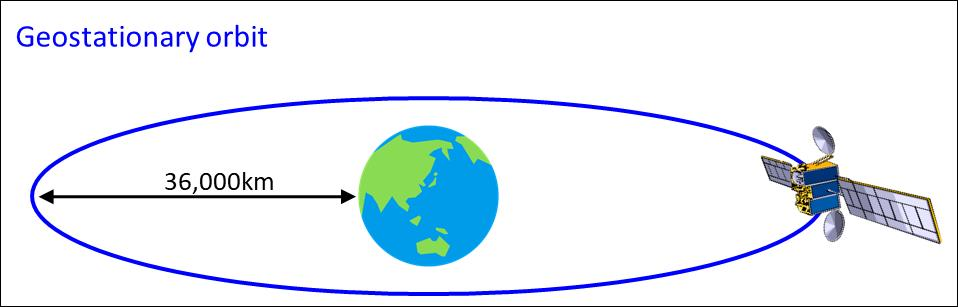QUESTION: 10

The acceleration due to gravity at the North Pole of Neptune is approximately 10.7 x  m/s2. Neptune has mass 1.0 x 1026 kg and radius 2.5 x 104 km and rotates once around its axis in about 16 h. What is the apparent weight a 5.0-kg object at Neptune’s equator?

Solution: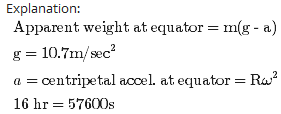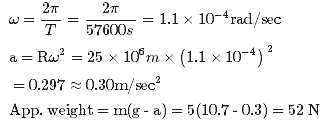QUESTION: 11

The force of attraction between a hollow spherical shell of uniform density and a point mass situated outside is just as if the entire mass of the shell is

Solution:
• According to Shell's theorem, If a particle of mass m is located outside a spherical shell of mass M at, for instance, point P, the shell attracts the particle as though the mass of the shell were concentrated at its centre.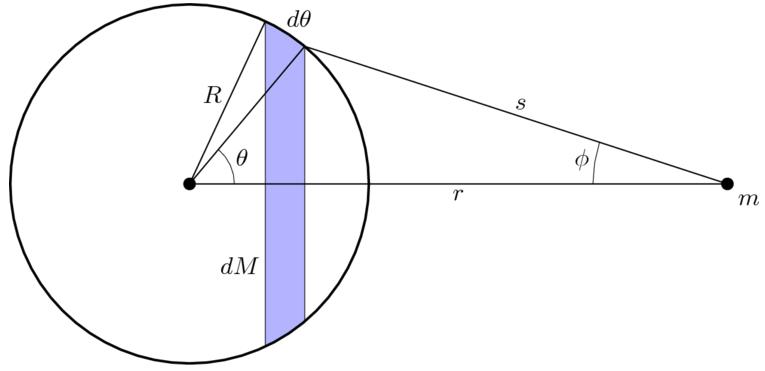• Thus, as far as the gravitational force acting on a particle outside the shell is concerned, a spherical shell acts no differently from the solid spherical distributions of mass.
QUESTION: 12

Geostationary Satellites

Solution:
• The orbital velocity of the satellite depends on its altitude above Earth.
• The nearer Earth, the faster the required orbital velocity. At an altitude of 200 kilometers, the required orbital velocity is just over 27,400 kph.
• To maintain an orbit that is 35,786 km above Earth, the satellite must orbit at a speed of about 11,300 kph. That orbital speed and distance permits the satellite to make one revolution in 24 hours.
• To achieve his speed, Judging from the mass of fuel needed, it stands to reason that you'd use a more powerful launch vehicle with a bi-propellant upper stage to get the satellite to GEO.
QUESTION: 13

The force of attraction due to a hollow spherical shell of uniform density, on a point mass situated inside it, is

Solution:
• The gravitational force acting by a spherically symmetric shell upon a point mass inside it, is the vector sum of gravitational forces acted by each part of the shell, and this vector sum is equal to zero.
• That is, a mass m within a spherically symmetric shell of mass M will feel no net force.
QUESTION: 14

Suppose there existed a planet that went around the sun twice as fast as the earth. What would be its orbital size as compared to that of the earth?

Solution:

Time period of revolution of earth around sun Te= 1 Year

Time period of revolution of planet around sun,Tp=0.5 Year

Orbital size of earth, re= 1 A.U

Orbital size of the planet,rp=?

Applying Kepler's third law we get: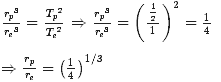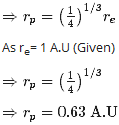QUESTION: 15

A particle of mass 3m is located 1.00 m from a particle of mass m. Where should you put a third mass M so that the net gravitational force on M due to the two masses is exactly zero?

Solution:

Let x be the distance of third particle from the mass 3m. then (1 - x) is the distance will be the distance from mass m. If we require that net force on another mass M be zero, then we must have the following :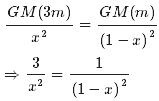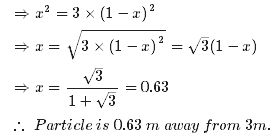QUESTION: 16

Moon has a mass of 7.36 x 1022 kg, and a radius of 1.74 x 106 m. Calculate the acceleration due to gravity on the moon.

Solution: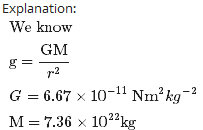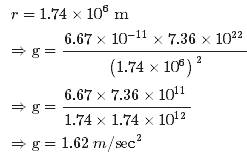QUESTION: 17

If a film of width l is stretched in the longitudinal direction a distance d by force F, surface tension is given by

Solution:
• Let L be the width of the film. Since the film has two surfaces, the total length along which the surface force acts on the slider is 2L.
• The surface tension S in the film is defined as the ratio of the surface force F to the length d (perpendicular to the force) along which the force acts S=F/d
• Hence, in the case, d = 2L S=F/2L
QUESTION: 18

Titania, the largest moon of the planet Uranus, has 1/8 the radius of the earth and 1/1700 the mass of the earth. What is the acceleration due to gravity at the surface of Titania

Data: G = 6.67x10−11 N m2/kg, RE = 6.38 x 106 m, mE = 5.97 x 1024 kg

Solution:

We know that gravitational acceleration, g = GM/R2
We know that M = Mass of earth /1700 and R = Radius of earth /8
Hence we get g = 64/1700 times the gravitational acceleration of earth
I.e. g = 64/1700 x 9.8
= 0.37 m/s2

QUESTION: 19

Which of the following statements correct?

Solution:

The acceleration due to gravity due to a body at point P on the surface of earth is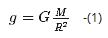Let the body is placed to point Q at a height h abovr the surface of earth, then acceleration due to gravity: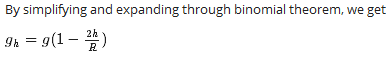From above expression we can clearly say that with increase in altitiude h , the value of acceleration due to gravity decreases.

QUESTION: 20

How will you ‘weigh the sun’, that is estimate its mass? The mean orbital radius of the earth around the sun is 1.5 x 108 km.

Solution:

R = Radius of Orbit of earth = 1.5 x 108 km = 1.5 x 1011m

T = time Period of earth around the sun = 365 Days